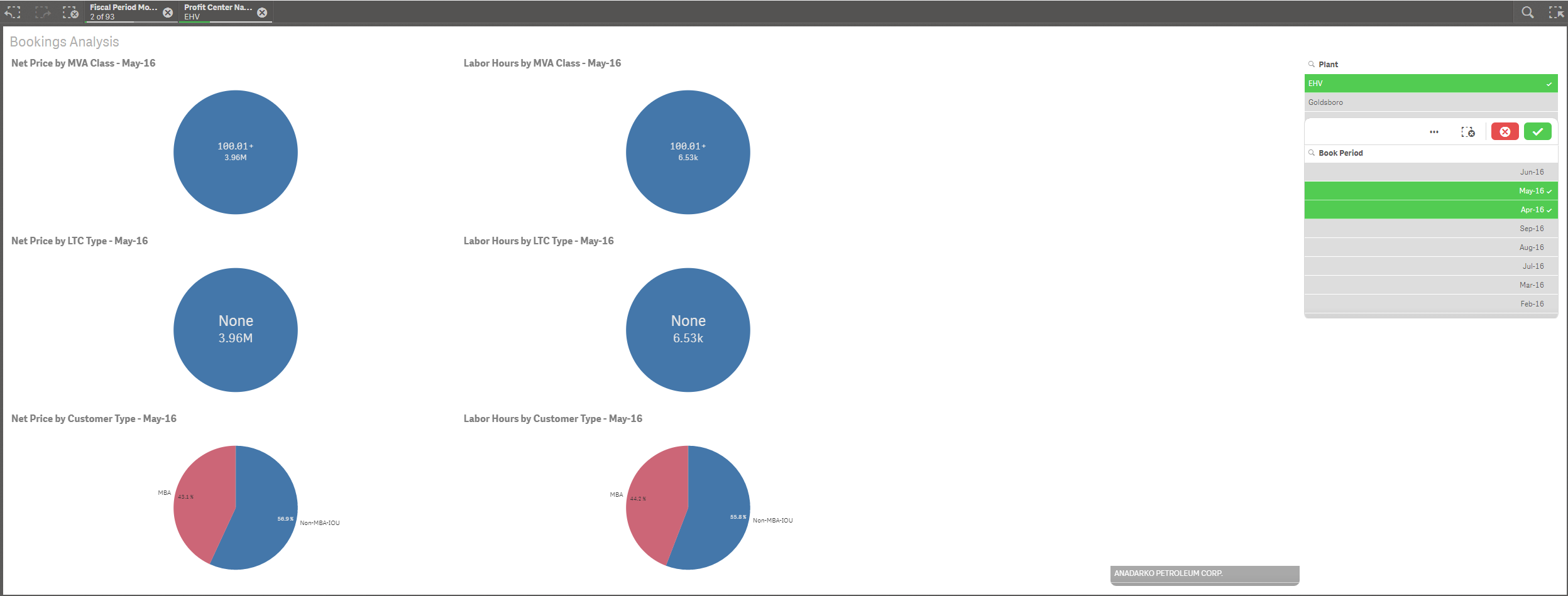# Qlik Sense App Development

Discussion board where members can learn more about Qlik Sense App Development and Usage.

Highlighted
New Contributor III

## How to modify set analysis to sum all periods selected?

Set analysis: Sum({<[Fiscal Period Counter Month] = {"\$(=max([Fiscal Period Counter Month]))"}>} [Sales])

This works perfectly for its intended purpose. However, when we make selections it defaults to the Max fiscal period month selected. How do I code it to use max month unless selections are made? I've tried a handful of IF statements but to no avail.

First image: June doesn't have an "EHV" sale so nothing is shown. Second image: Only April and May selected but only May sales are shown.Tags (2)
1 Solution

Accepted SolutionsMVP

## Re: How to modify set analysis to sum all periods selected?

Try maybe something like

=Sum(

{<[Fiscal Period Counter Month] *= {"\$(=If(GetSelectedCount([Fiscal Period Counter Month]),'*',max([Fiscal Period Counter Month])))"}>}

[Sales])

4 RepliesMVP

## Re: How to modify set analysis to sum all periods selected?

Try maybe something like

=Sum(

{<[Fiscal Period Counter Month] *= {"\$(=If(GetSelectedCount([Fiscal Period Counter Month]),'*',max([Fiscal Period Counter Month])))"}>}

[Sales])

New Contributor III

## Re: How to modify set analysis to sum all periods selected?

Thanks! I used a modified version of this using the GetSelectedCount function. Didn't know it existed before.MVP

## Re: How to modify set analysis to sum all periods selected?

You are welcome.

How does your modification look like?

New Contributor III

## Re: How to modify set analysis to sum all periods selected?

I had to expand on it cover several scenarios and added a variable,vPeriodCurrentMonth, to contain the original Set Analysis

if(GetSelectedCount([Fiscal Period Month Year])=0 and GetSelectedCount([Profit Center Name])=0 and GetSelectedCount([LTC Type])=0 and GetSelectedCount([Customer Name])=0 and GetSelectedCount([MVA Class])=0

,

Sum( \$(vPeriodCurrentMonth)

[Trans Sales]

)

+Sum( \$(vPeriodCurrentMonth)

[Freight Sales]

)

+Sum( \$(vPeriodCurrentMonth)

Rigging

),

Sum(

[Trans Sales]

)

+Sum(

[Freight Sales]

)

+Sum(

Rigging

))